8th Grade Math Home > Teacher Resources > Mathematical Power for All Students K-12 > Part III - Algebraic Thinking Strand

Algebraic Thinking Strand

Patterns

 Patterns allow us to generalize relationships within a set of data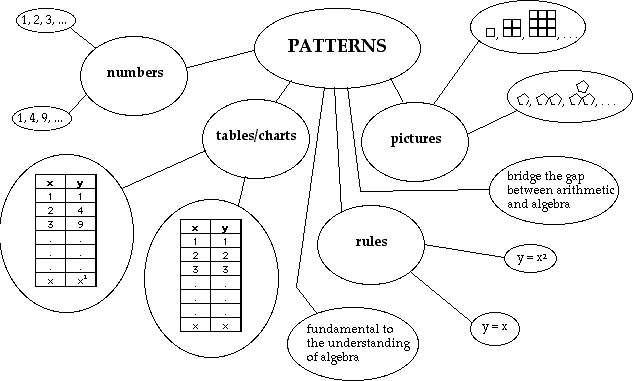Variables and Expressions

 A variable is a symbol used to represent a value.An expression is a mathematical phrase that uses numbers, variables, and/or operations.Equations and Inequalities

 An equation is a sentence that has two equal mathematical expressions.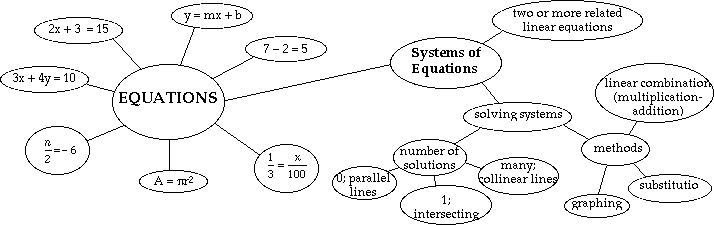An inequality is any mathematical sentence that is not an equation.Functions

 A function is a relationship in which the value of one variable depends on the value of another variable; each first value may be paired with one and only one second value.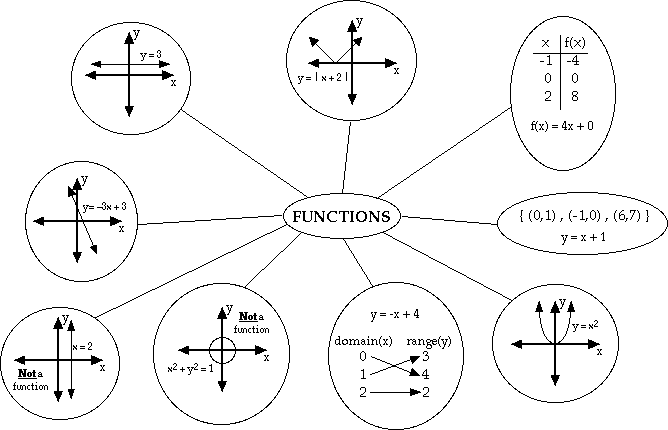Ratios, Rates, and Proportions

 A ratio is a comparison of two of the same measurements.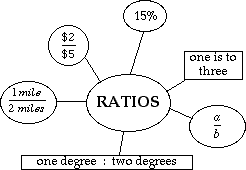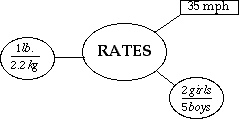A rate is a comparison of two different measurements.
 A proportion is a comparison of two equivalent ratios.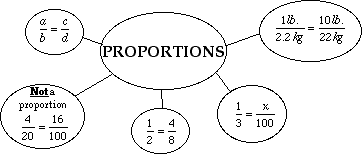Multiple Representations

 A single mathematical concept can be represented in a variety of forms such as symbols, words, graphs, tables, and equations ...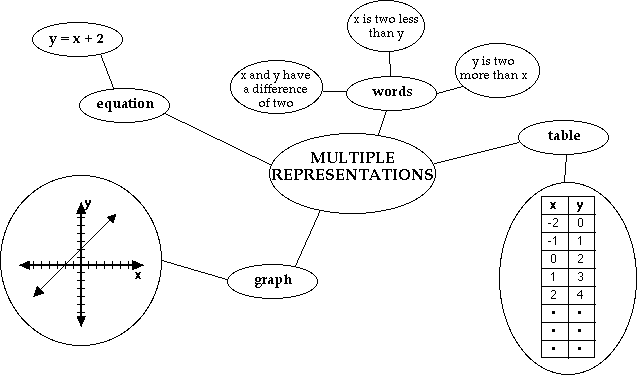Data Analysis

 The collection and organization of information into a form that is easily understood.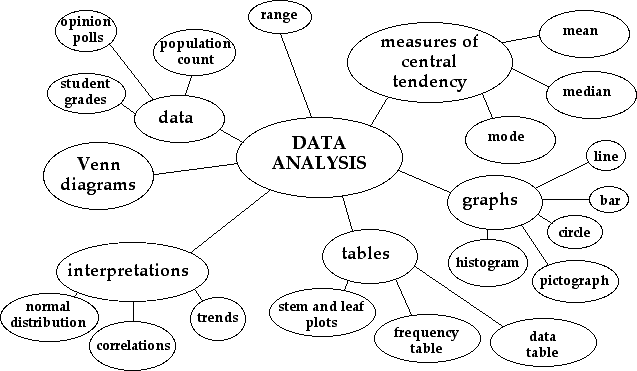8th Grade Math Home > Teacher Resources > Mathematical Power for All Students K-12 > Part III - Algebraic Thinking Strand